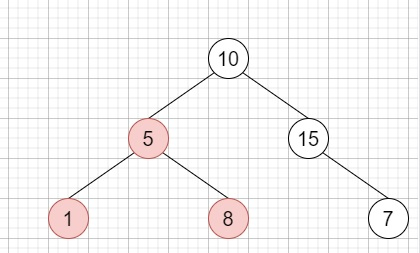# Largest BST Subtree in C++

Suppose we have a binary tree; we have to find the largest subtree of it where largest means subtree with largest number of nodes in it.

So, if the input is like,then the output will be 3, as the Largest BST Subtree, in this case, is the highlighted one.

To solve this, we will follow these steps −

• Define one structure called data, there will be four values, size, maxVal, minVal, and ok, the ok can hold only true/false values

• solve(TreeNode * node)

• if node is null, then &miuns;

• return Data by initializing (0, infinity, -infinity, true)

• left := solve(left of node)

• left := solve(right of node)

• Define one data called curr

• curr.ok := false

• if val of node >= right.minVal, then −

• return curr

• if val of node <= left.maxVal, then −

• return curr

• if left.ok is true and right.ok is true, then −

• curr.sz := 1 + left.sz + right.sz

• curr.ok := true

• curr.maxVal := maximum of (val of node and right.maxVal)

• curr.minVal := maximum of (val of node and left.minVal)

• if curr.ok is true, then −

• ret := maximum of ret and curr.sz

• return curr

• From the main method, do the following −

• ret := 0

• solve(root)

• return ret

## Example

Let us see the following implementation to get a better understanding −

Live Demo

#include <bits/stdc++.h>
using namespace std;
class TreeNode{
public:
int val;
TreeNode *left, *right;
TreeNode(int data){
val = data;
left = NULL;
right = NULL;
}
};
void insert(TreeNode **root, int val){
queue<TreeNode*> q;
q.push(*root);
while(q.size()){
TreeNode *temp = q.front();
q.pop();
if(!temp->left){
if(val != NULL)
temp->left = new TreeNode(val);
else
temp->left = new TreeNode(0);
return;
}
else{
q.push(temp->left);
}
if(!temp->right){
if(val != NULL)
temp->right = new TreeNode(val);
else
temp->right = new TreeNode(0);
return;
}
else{
q.push(temp->right);
}
}
}
TreeNode *make_tree(vector<int< v){
TreeNode *root = new TreeNode(v);
for(int i = 1; i<v.size(); i++){
insert(&root, v[i]);
}
return root;
}
struct Data{
int sz;
int maxVal;
int minVal;
bool ok;
Data(){}
Data(int a, int b, int c, bool d){
sz = a;
minVal = b;
maxVal = c;
ok = d;
}
};
class Solution {
public:
int ret;
Data solve(TreeNode* node){
if (!node)
return Data(0, INT_MAX, INT_MIN, true);
Data left = solve(node->left);
Data right = solve(node->right);
Data curr;
curr.ok = false;
if (node->val >= right.minVal) {
return curr;
}
if (node->val <= left.maxVal) {
return curr;
}
if (left.ok && right.ok) {
curr.sz = 1 + left.sz + right.sz;
curr.ok = true;
curr.maxVal = max(node->val, right.maxVal);
curr.minVal = min(node->val, left.minVal);
}
if (curr.ok)
ret = max(ret, curr.sz);
return curr;
}
int largestBSTSubtree(TreeNode* root){
ret = 0;
solve(root);
return ret;
}
};
main(){
Solution ob;
vector<int< v = {10,5,15,1,8,NULL,7};
TreeNode *root= make_tree(v);
cout << (ob.largestBSTSubtree(root));
}

## Input

[10,5,15,1,8,null,7]

## Output

3